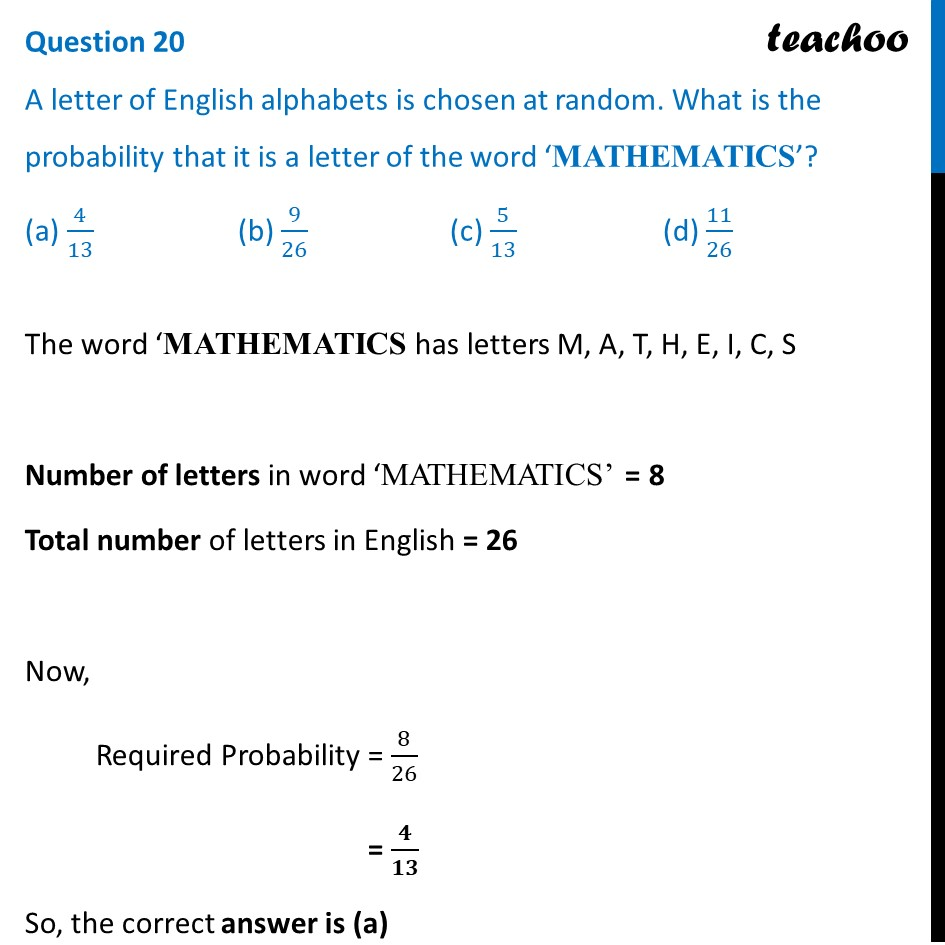## (a) 4/13  (b) 9/26   (c) 5/13   (d) 11/261. Class 10
2. Solutions of Sample Papers for Class 10 Boards
3. CBSE Class 10 Sample Paper for 2022 Boards - Maths Standard [MCQ]

Transcript

Question 20 A letter of English alphabets is chosen at random. What is the probability that it is a letter of the word ‘MATHEMATICS’? (a) 4/13 (b) 9/26 (c) 5/13 (d) 11/26 The word ‘MATHEMATICS has letters M, A, T, H, E, I, C, S Number of letters in word ‘MATHEMATICS’ = 8 Total number of letters in English = 26 Now, Required Probability = 8/26 = 𝟒/𝟏𝟑 So, the correct answer is (a)